### Fractions - Solutions

CBSE Class –VI Mathematics

NCERT Solutions
Chaper 7 Fraction (Ex. 7.1)

Question 1. Write the fraction representing the shaded portion: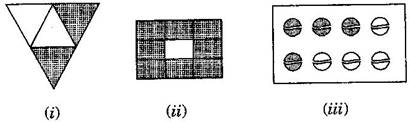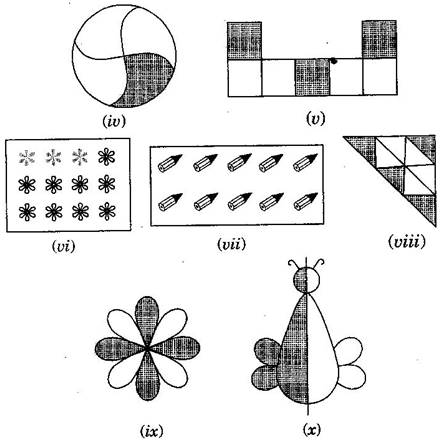Answer: (i)$\frac{2}{4}$ (ii) $\frac{8}{9}$ (iii) $\frac{4}{8}$ (iv)$\frac{1}{4}$ ( v)$\frac{3}{7}$

(vi)$\frac{9}{12}$ (vii)$\frac{10}{10}$ (viii)$\frac{4}{9}$ (ix)$\frac{4}{8}$ (x)$\frac{1}{2}$

Question 2. Colour the part according to the given fraction: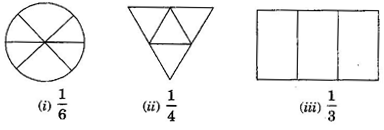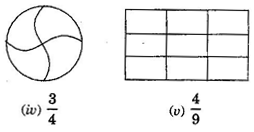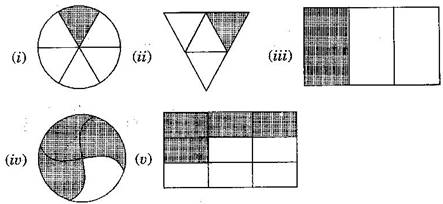Question 3. Identify the error, if any?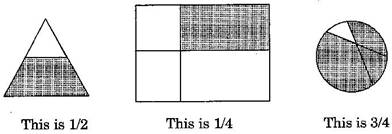Answer: All the figures are not equally divided. For making fractions, it is necessary that figure is divided into equal parts.

Question 4. What fraction of a day is 8 hours?

Answer: Since, 1 day = 24 hours.

Therefore, the fraction of 8 hours = $\frac{8}{24}=\frac{1}{3}$

Question 5. What fraction of an hour is 40 minutes?

Answer: Since, 1 hour = 60 minutes.

Therefore, the fraction of 40 minutes = $\frac{40}{60}=\frac{2}{3}$

Question 6. Arya, Abhimanyu and Vivek shared lunch. Arya has brought two sandwiches, one made of vegetable and one of jam. The other two boys forgot to bring their lunch. Arya agreed to share his sandwiches so that each person will have an equal share of each sandwich.

(a)How can Arya divide his sandwiches so that each person has an equal share?

(b)What part of a sandwich will each boy receive?

Answer:(a) Arya will divide each sandwich into three equal parts and give one part of each sandwich to each one of them.

(b) $1×\frac{1}{3}=\frac{1}{3}$

Question 7. Kanchan dyes dresses. She had to dye 30 dresses. She has so far finished 20 dresses. What fraction of dresses has she finished?

Answer: Total number of dresses to dye= 30

Work completed = 20

Fraction of completed work = $\frac{20}{30}=\frac{2}{3}$

Question 8. Write the natural numbers from 2 to 12. What fraction of them are prime numbers?

Answer: Natural numbers from 2 to 12: 2, 3, 4, 5, 6, 7, 8, 9, 10, 11, 12

Prime numbers from 2 to 12: 2, 3, 5, 7, 11

Hence, fraction of prime numbers = $\frac{5}{11}$

Question 9. Write the natural numbers from 102 to 113. What fraction of them are prime numbers?

Answer: Natural numbers from 102 to 113: 102, 103, 104, 105, 106, 107, 108, 109, 110, 111, 112, 113

Prime numbers from 102 to 113: 103, 107, 109, 113

Hence fraction of prime numbers = $\frac{4}{12}=\frac{1}{3}$

Question 10. What fraction of these circles has ‘X’s in them?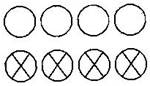Answer: Total number of circles = 8 and number of circles having ‘X’ = 4

Hence, the fraction = $\frac{4}{8}$

Question 11. Kristin received a CD player for her birthday. She bought 3 CDs and received 5 others as gifts. What fraction of her total CDs did she buy and what fraction did she receive as gifts?

Answer: Total number of CDs = 3 + 5 = 8

Number of CDs purchased = 3

Fraction of CDs purchased = $\frac{3}{8}$